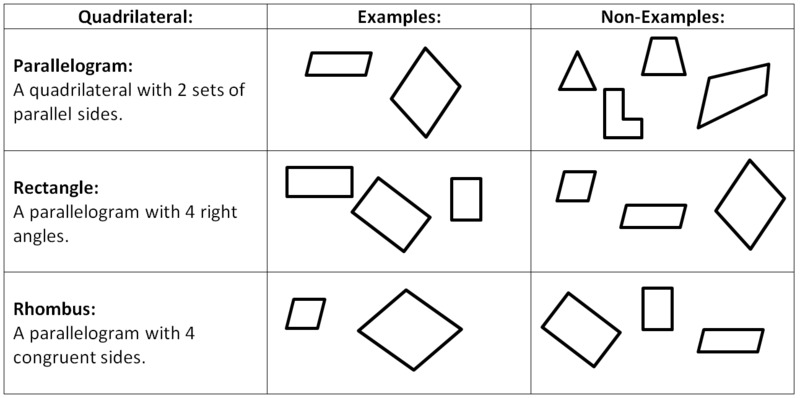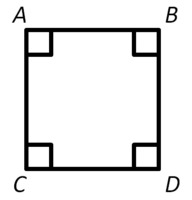# What shape am I?

Alignments to Content Standards: 4.G.A.2

1. Draw at least two examples and two non-examples of each of the quadrilaterals defined below.

• Parallelogram: A quadrilateral with 2 pairs of parallel sides.
• Rectangle: A parallelogram with 4 right angles.
• Rhombus: A parallelogram with 4 sides with equal length.

2. I am a shape that is a parallelogram, a rectangle, and at the same time a rhombus. What shape am I? Draw a diagram of what I look like. Use the vocabulary words and their definitions given in part (a) to explain what shape I am.

## IM Commentary

This task builds on the geometric knowledge that students developed in 2nd grade (2.G.1). In this task, students ultimately use the definitions they are given for three types of quadrilaterals and what they know about parallel sides to identify that a square fits all the definitions and explain why. By drawing examples and non-examples for each kind of shape, students get an opportunity to explore their individual properties before relating their knowledge of all three to the defining attributes of a square.

Students should be encouraged to work in small groups and use the correct vocabulary as they talk together about classifying shapes. Students should take care to draw their figures with a straight-edge so that they can draw precise shapes. It is a good idea to have a whole-group discussion about this task to be sure that students understand the relationship between these different quadrilaterals.

For students who need more scaffolding, the teacher can provide a bank of shapes for them to sort as “examples” and “non-examples” in part (a). These can be cut-outs or graphics on the page, depending on the needs of the students. A graphic organizer would help students to summarize their thinking from this task.

## Solution

1. There are many possible examples and non-examples; the figure below shows a few.2. A square has two pairs of parallel sides, therefore it is a parallelogram; in the figure, we see that $\overline{AB}$ is parallel to $\overline{CD}$ and $\overline{AC}$ is parallel to $\overline{BD}$. A square also has 4 right angles, so therefore it is a rectangle. A square also has 4 congruent sides, therefore it is a rhombus.3. Since all rectangles and all rhombuses are parallelograms, and all shapes that are both rectangles and rhombuses are also parallelograms, we can show the relationship between these figures with this Venn diagram: